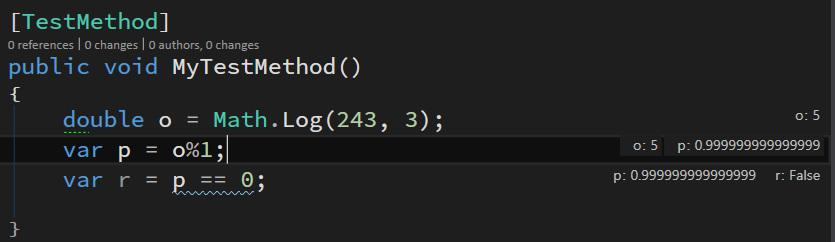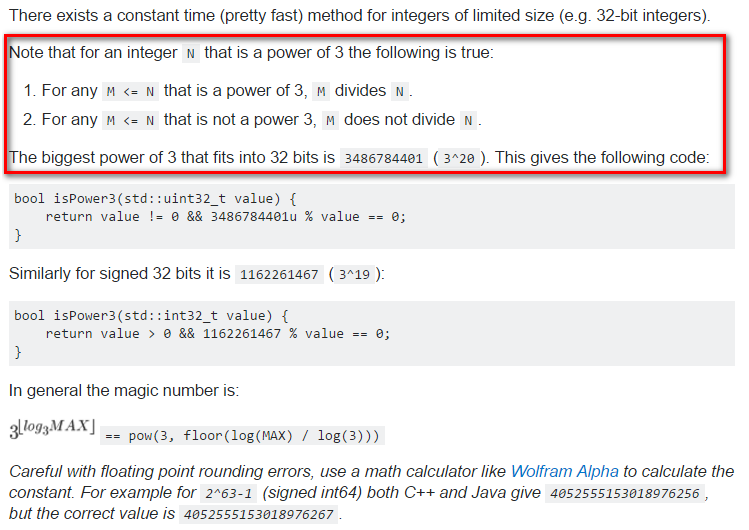### LeetCode 326. Power of Three

LeetCode 326: Power of Three

Given an integer, write a function to determine if it is a power of three.

Could you do it without using any loop / recursion?

## 沒啥想法？先寫一個測試案例出來就對了。

        [TestMethod]
public void n_is_3_should_be_true()
{
var n = 3;
Assert.IsTrue(new Solution().IsPowerOfThree(n));
}

## 產品代碼思路

1. n 除以 3, 若餘數不為 0，則 return false;
2. 若餘數為 0，則商數當下一次的 n，再次除以 3 運算。

...
...
...沒有，這算法就是得迴圈或遞迴## 如何判斷一個 double 是否為整數

    public class Solution
{
public bool IsPowerOfThree(int n)
{
var o = Math.Log(n, 3);

var p = o % 1;

return p == 0;
}
}

## Double 精準度問題public bool IsPowerOfThree(int n)
{
var o = (decimal)Math.Log(n, 3);

var r = o % 1;

return r == 0;
}

## 當 n = 0 會 overflow exception

Math.Log() 當傳入 n 為 = 0 時，會拋 overflow exception，因為 n 只要是整數都可以傳入。所以直接針對 n 的範圍作限制與判斷。產品代碼如下：

    public class Solution
{
public bool IsPowerOfThree(int n)
{
if (n <= 0) return false;

var o = (decimal)Math.Log(n, 3);

var r = o % 1;

return r == 0;
}
}

## 重構：inline variable

    public class Solution
{
public bool IsPowerOfThree(int n)
{
if (n <= 0) return false;

return (decimal)Math.Log(n, 3) % 1 == 0;
}
}

## 更新想收到第一手公開培訓課程資訊，或想詢問企業內訓、顧問、教練、諮詢服務的，請洽 Facebook 粉絲專頁：91敏捷開發之路。